# Texas Go Math Grade 7 Module 11 Answer Key Analyzing and Comparing Data

Refer to our Texas Go Math Grade 7 Answer Key Pdf to score good marks in the exams. Test yourself by practicing the problems from Texas Go Math Grade 7 Module 11 Answer Key Analyzing and Comparing Data.

## Texas Go Math Grade 7 Module 11 Answer Key Analyzing and Comparing Data

Write each fraction as a decimal and a percent.

Question 1.
$$\frac{7}{8}$$ _____
Divide 7 by 8. Write a decimal point and zeros in the dividend and place a decimal point in the quotient.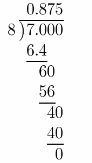The result is: 0.875
Move the decimal point two places to the right and add the “%“ sign.
0.875 = 87.5%
$$\frac{7}{8}$$ = 87.5%

$$\frac{4}{5}$$ _____
Divide 4 by 5. Write a decimal point and zeros in the dividend and place a decimal point in the quotient.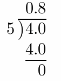The result is: 0.8 = 0.800
Move the decimal point two places to the right and add the “%” sign.
0.8 = 80%
$$\frac{4}{5}$$ = 80%

Question 3.
$$\frac{4}{5}$$ _____
Divide 1 by 4. Write a decimal point and zeros in the dividend and place a decimal point in the quotient.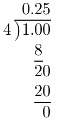The result is: 0.25
Move the decimal point two places to the right mid add the”%” sign.
0.25 = 25%
$$\frac{1}{4}$$ = 25%

Question 4.
$$\frac{3}{10}$$ _____
Divide 3 by 10. Write a decimal point, and zeros in the dividend and place a decimal point in the quotient.The result is: 0.3 = 0.300
Move the decimal point two places to the right and add the “%” sign.
0.3 = 0.300 = 30%
$$\frac{3}{10}$$ = 30%

Grade 7 Math Module 11 Answer Key Analyzing and Comparing Data Question 5.
$$\frac{19}{20}$$ _____
Divide 19 by 20. Write a decimal point and zeros in the dividend and place a decimal point in the quotient.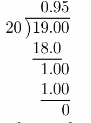The result is: 0.95
Move the decimal point two places to the right and add the “ %“ sign.
0.95 = 95%
$$\frac{19}{20}$$ = 95%

Question 6.
$$\frac{7}{25}$$ _____
Divide 7 by 25. Write a decimal point and zeros in the dividend and place a decimal point in the quotient.The result is: 0.28
Move the decimal point two places to the right and add the ”%” sign.
0.28 = 28%
$$\frac{7}{25}$$ = 28%

Question 7.
$$\frac{37}{50}$$ _____
Divide 37 by 50. Write a decimal point and zeros in the dividend and place a decimal point in the quotient.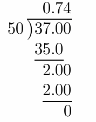The result is: 0.74
Move the decimal point two places to the tile right awl add the %“ sign.
0.74 = 74%
$$\frac{37}{50}$$ = 74%

$$\frac{29}{100}$$ _____
Divide 29 by 100. Write a decimal point and zeros in the dividend and place a decimal point in the quotient.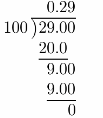The result is: 0.29
Move the decimal point two places to the right and add the “%“ sign.
0.29 = 29%
$$\frac{29}{100}$$ = 29%

Find the median and the mode of the data.

Question 9.
11, 17, 7, 6, 7, 4, 15, 9 _________
Put number in order centering 4, 6, 7, 7, 9, 11, 15, 17
Median is the middle number; found by ordering alt data points and picking out the one in the middle. There are three middle numbers, so take the mean of those three numbers).
centering Median = $$\frac{6+7+11}{3}$$ = $$\frac{24}{3}$$ = 8
centering Mode is the number that occurs the highest number of times.
Mode = 7
centering Median= 8
centering Mode= 7

Question 10.
43, 37, 49, 51, 56, 40, 44, 50, 36 _____
Put number in order
centering 36, 37, 40, 43, 44, 49, 50, 51, 56
Median is the middle number; found by ordering all, data points and picking out the one in the middle. There are
four middle numbers,so take the mean of those four numbers.
Median = $$\frac{37+43+49+51}{4}$$ = $$\frac{180}{4}$$ = 45
centering Mode is the number that occurs the highest number of times.
Mode: All numbers only appear.

Median= 45
Mode: All numbers only appear once.

Find the mean of the data.

Question 11.
9, 16, 13, 14, 10, 16, 17,9 ________
Mean: found by adding all data points and dividing by the number of data points.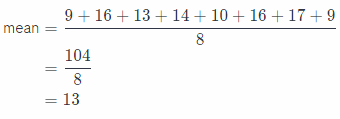mean = 13

Analyzing and Comparing Data Module 11 Grade 7 Answer Key Question 12.
108, 95, 104, 96, 97, 106, 94 ________
Mean: found by adding all data points and dividing by the number of data points.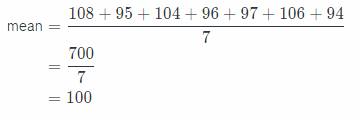mean = 100

Visualize Vocabulary
Use the ✓ words to complete the right column of the chart.Complete each sentence using the preview words.

Question 1.
A display that uses values from a data set to show how the values are spread out is a ____.